# 机器学习之决策树(四)

By | 2018年7月19日

1 决策树概述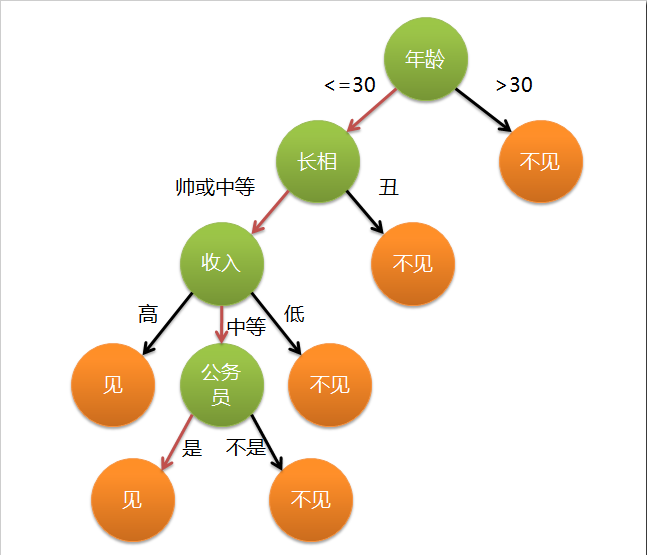2 决策树分类

3 构造决策树

1、属性是离散值且不要求生成二叉决策树。此时用属性的每一个划分作为一个分支。

2、属性是离散值且要求生成二叉决策树。此时使用属性划分的一个子集进行测试，按照“属于此子集”和“不属于此子集”分成两个分支。

3、属性是连续值,此时确定一个值作为分裂点split_point，按照>split_point和<=split_point生成两个分支

4 ID3算法

ID3算法就是在每次需要分裂时，计算每个属性的增益率，然后选择增益率最大的属性进行分裂

(1) 计算公式: p(x)是选择该分类的概率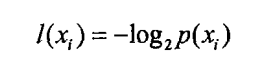(2) 为了计算熵，我们需要计算所有类别所有可能值包含的信息期望值，通过下面的公式得到：

n是分类的数目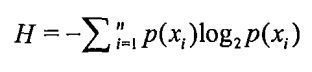(3) 在决策树当中，设D为用类别对训练元组进行的划分，则D的熵（entropy）表示为：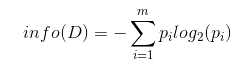(4) 现在我们假设将训练元组D按属性A进行划分，则A对D划分的期望信息为: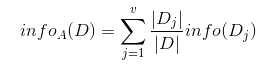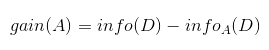ID3算法就是在每次需要分裂时，计算每个属性的增益率，然后选择增益率最大的属性进行分裂。下面我们继续用SNS社区中不真实账号检测的例子说明如何使用ID3算法构造决策树。我们假设训练集合包含10个元素：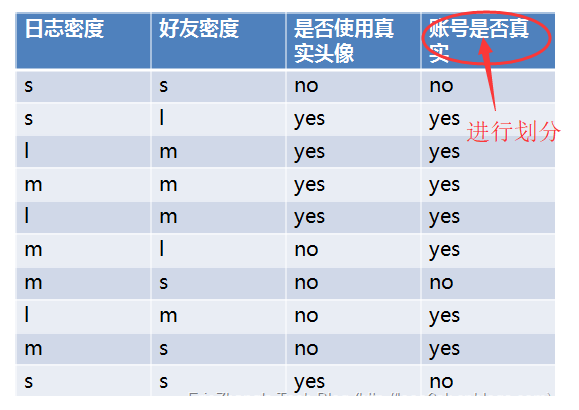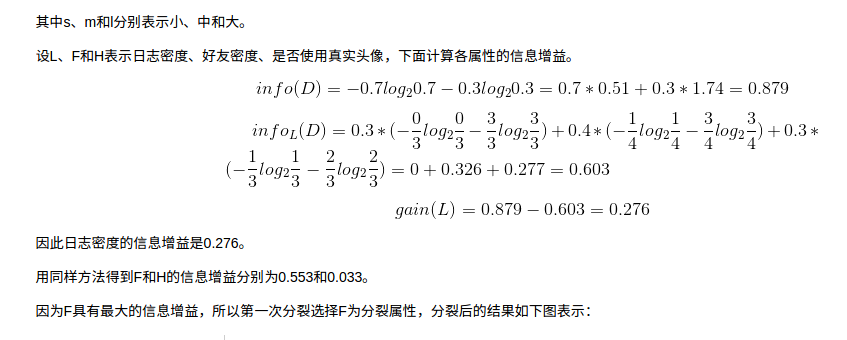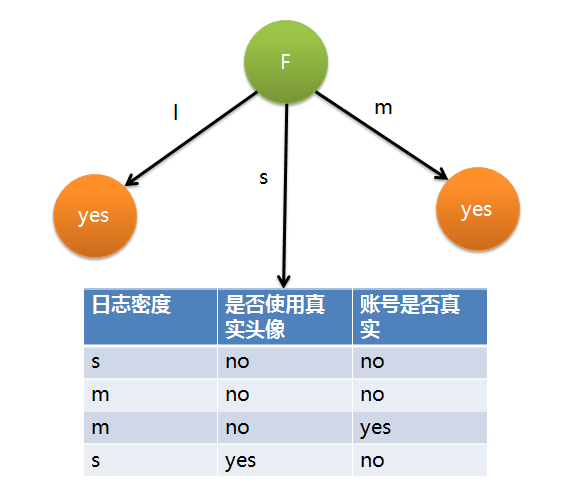``````import numpy as np
import pandas as pd
from pandas import Series,DataFrame
import math
# no  p = 3/10  0.3
# yes p = 0.7

# 按照类别对训练数据进行的划分
# 数据样本原始的熵
info_D = -0.3*math.log2(0.3) -0.7*math.log2(0.7)
info_D
#输出  0.8812908992306927``````

``````# s  0.3  no yes no    -- no 2/3  yes 1/3
# l  0.3  yes yes yes  -- no 0    yes 1
# m  0.4  yes yes no yes -- no 1/4 yes 3/4

info_L_D = 0.3*(-2/3*math.log2(2/3)-1/3*math.log2(1/3)) + 0.3*(-1*math.log2(1)) + 0.4*(-1/4*math.log2(1/4)-3/4*math.log2(3/4))
info_L_D
#输出  0.6
info_D - info_L_D
#输出  0.2812908992306927``````

``````# 计算按照好友密度划分的信息熵
# s 0.4  no no yes no   --- no 3/4 yes 1/4
# l 0.2  yes yes        --- no 0   yes 1
# m 0.4  yes yes yes yes -- no 0   yes 1
info_F_D = 0.4*(-3/4*math.log2(3/4)-1/4*math.log2(1/4))
info_F_D
#输出  0.32451124978365314
info_D - info_F_D
#输出  0.5567796494470396``````

``````# no  0.5  no yes no yes yes   ---  no 2/5  yes 3/5
# yes 0.5  yes yes yes yes no  ---  no 1/5  yes 4/5
info_H_D = 0.5*(-2/5*math.log2(2/5)-3/5*math.log2(3/5)) + 0.5*(-1/5*math.log2(1/5)-4/5*math.log2(4/5))
info_H_D
#输出 0.8464393446710154
info_D - info_H_D
#输出 0.034851554559677256``````

1 比较KNN 逻辑斯蒂 决策树进行分类

``````from sklearn import datasets
from sklearn.tree import DecisionTreeClassifier
from sklearn.neighbors import KNeighborsClassifier
from sklearn.linear_model import LogisticRegression

#导入IRIS数据集,加载IRIS的数据集
X = iris.data
y = iris.target

#max_depth 设置决策树的最大深度
reg1 = DecisionTreeClassifier(max_depth=5)
reg1.fit(X,y).score(X,y)  #输出  1.0
#KNN
reg2 = KNeighborsClassifier()
reg2.fit(X,y).score(X,y)  #输出0.967
#逻辑斯蒂
reg3 = LogisticRegression()
reg3.fit(X,y).score(X,y)  #输出0.96``````

2 预测一个椭圆

``````#导包
import numpy as np
import matplotlib.pyplot as plt
from sklearn.tree import DecisionTreeRegressor

# 创建X与y
rng = np.random.RandomState(1) #伪随机数 == np.random.seed(1) 可预知的随机数

#随机生成-100 到100的数字,这些数字就是角度
X = np.sort(200 * rng.rand(100,1) - 100,axis = 0)
#根据角度生成正弦值和余弦值，这些值就是圆上面的点
y = np.array([np.pi * np.sin(X).ravel(),np.pi * np.cos(X).ravel()]).transpose()
#添加噪声
y[::5,:] += (0.5 -rng.rand(20,2))

#参数max_depth越大，越容易过拟合
# 第1步：训练
regr1 = DecisionTreeRegressor(max_depth=2)
regr2 = DecisionTreeRegressor(max_depth=5)
regr3 = DecisionTreeRegressor(max_depth=8)

regr1.fit(X,y)
regr2.fit(X,y)
regr3.fit(X,y)

# 第2步：预测
X_test = np.arange(-100.0,100.0,0.01)[:,np.newaxis]
y_1 = regr1.predict(X_test)
y_2 = regr2.predict(X_test)
y_3 = regr3.predict(X_test)``````

``````# 显示图像
plt.figure(figsize=(12,8))
s = 50

plt.subplot(221)
plt.scatter(y[:,0],y[:,1],c='navy',s=s,label='data')
plt.legend()

plt.subplot(222)
plt.scatter(y_1[:,0],y_1[:,1],c='b',s=s,label='data')
plt.legend()

plt.subplot(223)
plt.scatter(y_2[:,0],y_2[:,1],c='r',s=s,label='data')
plt.legend()

plt.subplot(224)
plt.scatter(y_3[:,0],y_3[:,1],c='g',s=s,label='data')
plt.legend()

plt.show()``````3 下面我们对鸢尾花的案例再次进行分析

``````from sklearn.tree import DecisionTreeClassifier
from sklearn.neighbors import KNeighborsClassifier
from sklearn.linear_model import LogisticRegression
#导包加载数据
from sklearn.datasets import load_iris
data = iris.data
target = iris.target``````
``````# 特征选择？？ 方差选择
data.std(axis=0)
# array([0.82530129, 0.43214658, 1.75852918, 0.76061262])
train = data[:,[0,3]]
import matplotlib.pyplot as plt
%matplotlib inline
plt.scatter(train[:,0],train[:,1],c=target)````````````# 获取预测集（屏幕中所有的点）
xmin,xmax = train[:,0].min(),train[:,0].max()
ymin,ymax = train[:,1].min(),train[:,1].max()
x = np.linspace(xmin,xmax,300)
y = np.linspace(ymin,ymax,300)
#meshgrid函数用两个坐标轴上的点在平面上画格。
xx,yy = np.meshgrid(x,y)
X_test = np.c_[xx.ravel(),yy.ravel()]``````

``````# max_depth 决策树深度，一般设置的值不要超过特征数量
# max_depth 值越大，拟合度越高
tree = DecisionTreeClassifier(max_depth=1)
tree.fit(train,target)``````

``````knn = KNeighborsClassifier(n_neighbors=3)
knn.fit(train,target)``````

``````logistic = LogisticRegression()
logistic.fit(train,target)``````

``results = [tree.predict(X_test),knn.predict(X_test),logistic.predict(X_test)]``

``````from matplotlib.colors import ListedColormap
cmap = ListedColormap(['#00aaff','#ff00aa','#aaff00'])

titles = ['DecisonTree','Knn','Logistic']
plt.figure(figsize=(12,3))
for i,y_ in enumerate(results):
axes = plt.subplot(1,3,i+1)
axes.scatter(X_test[:,0],X_test[:,1],c=y_,cmap=cmap)
axes.set_title(titles[i])
axes.scatter(train[:,0],train[:,1],c=target)``````via:https://www.cnblogs.com/why957/p/9337896.html